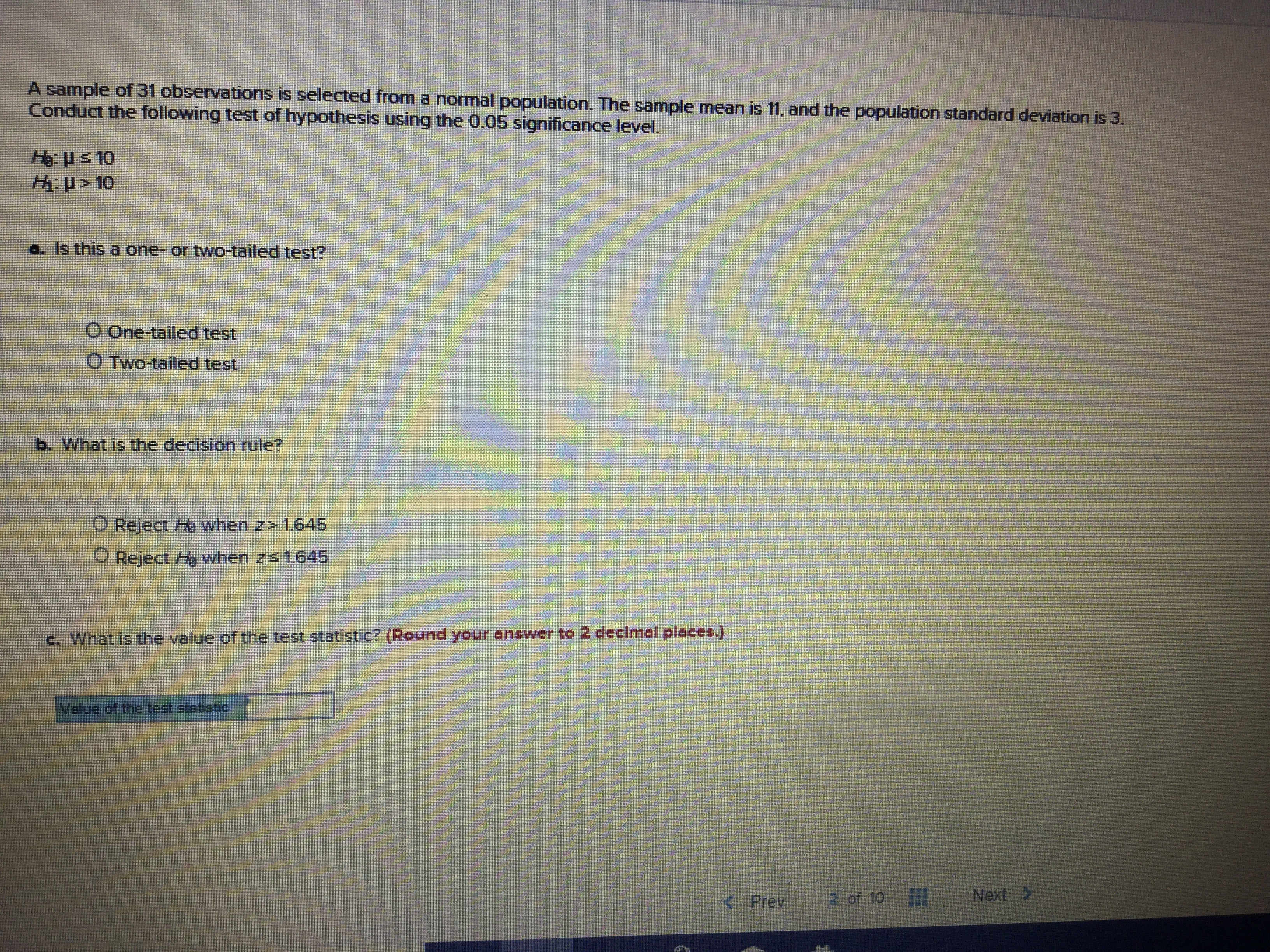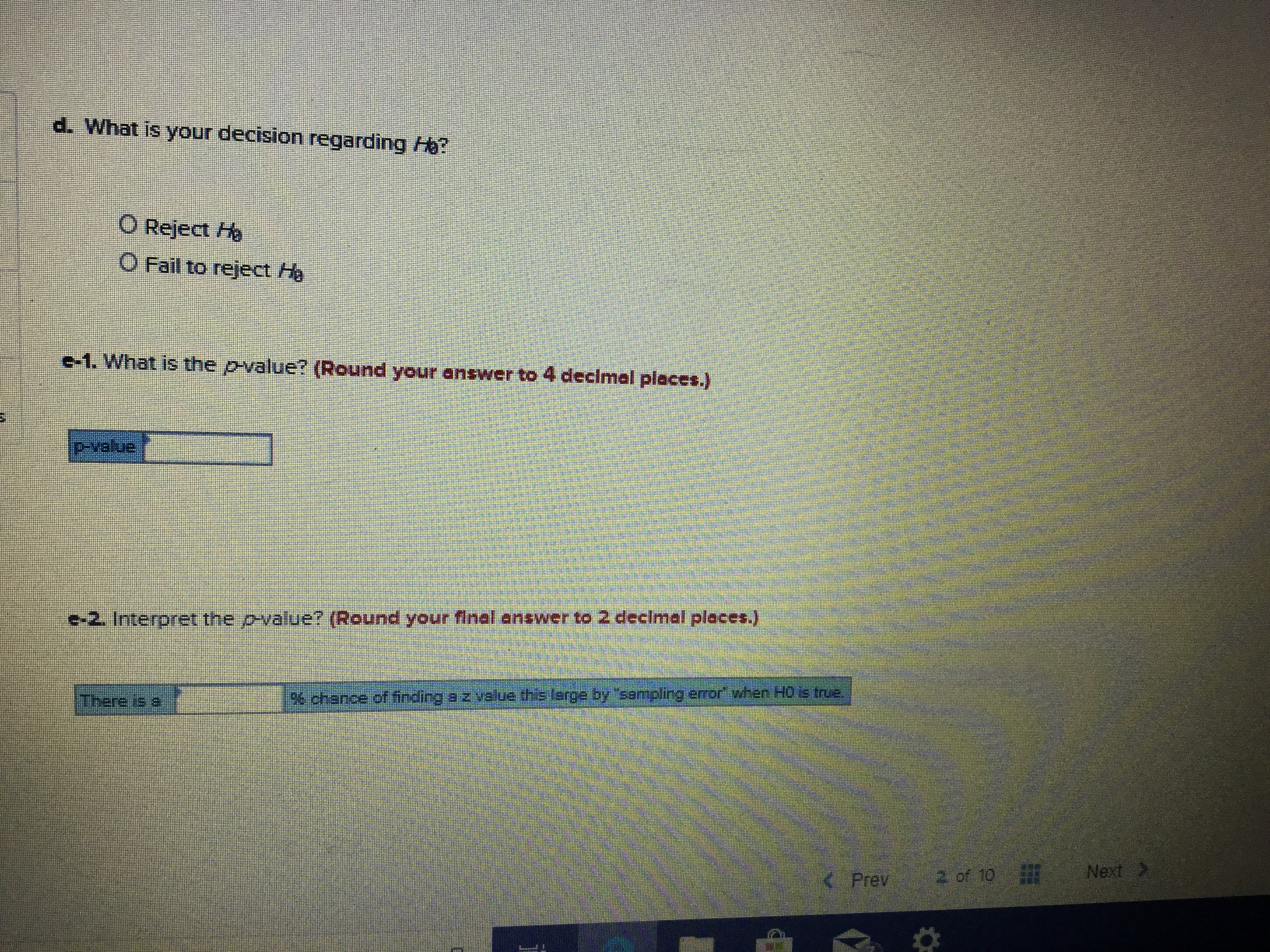# A sample of 31 observations is selected from a normal populaticon. The sample mean is 11, and the population standard deviation is 3. Conduct the following test of hypothesis using the 0.05 significance level. Hus 10 H>10 a. Is this a one- or two-tailed test? o One-tailed test O Two-tailed test b. What is the decision rule? O Reject H when z> 1.645 when 2s 1645 Reject c. What is the value of the test statistic? (Round your answer to 2 decimal places.) Value of the test statistic 2 of 10 Next> 2 of 10 KPrev

Question

I would like the solutions for each part of the problemhelp_outlineImage TranscriptioncloseA sample of 31 observations is selected from a normal populaticon. The sample mean is 11, and the population standard deviation is 3. Conduct the following test of hypothesis using the 0.05 significance level. Hus 10 H>10 a. Is this a one- or two-tailed test? o One-tailed test O Two-tailed test b. What is the decision rule? O Reject H when z> 1.645 when 2s 1645 Reject c. What is the value of the test statistic? (Round your answer to 2 decimal places.) Value of the test statistic 2 of 10 Next>help_outlineImage Transcriptionclosed. What is your decision regarding H? O Reject Ho O Fail to reject Ha -1. What is the pvalue? (Round your answer to 4 declmal places.) pvallue 2. Interpret the p-value? (Round your final enswer to 2 decimal places.) There is a Be chance of fincling a z velue this large by sompling error when H0 s true. Next> 2 of 10 KPrev fullscreen

### Want to see this answer and more?

Experts are waiting 24/7 to provide step-by-step solutions in as fast as 30 minutes!*

*Response times may vary by subject and question complexity. Median response time is 34 minutes for paid subscribers and may be longer for promotional offers.
Tagged in
Math
Statistics

### Hypothesis Testing